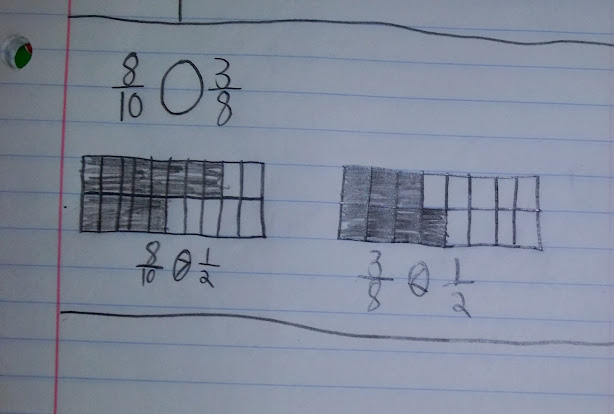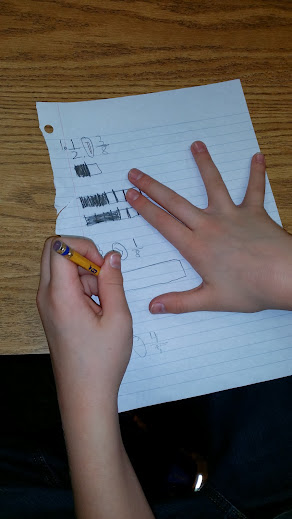# Close to 0, 1/2, or 1?

1 teachers like this lesson
Print Lesson

## Objective

SWBAT compare two fractions using a benchmark such as 1/2.

#### Big Idea

Is it closer to 0, 1/2 or 1? Students will have to look carefully when using this direct instruction approach. Students model fractions compared with 1/2 in order to compare fractions.

## Warm Up

15 minutes

In this lesson students use the benchmark fraction of 1/2, however, in future lessons students work with other benchmark fractions - 1/4, 1/3, 1/2, 2/3, 3/4.

I begin this warm up by asking students if they have ever heard of a benchmark.  I ask, "How could we use 50 as a benchmark?  Or 100 as a benchmark?"  I lead a discussion about the meaning of benchmark—something we know or understand that can be used to help us estimate something—and that sometimes we can associate a number with a benchmark and sometimes it’s just a comparison.

One example I use is I ask students how they would explain to a little kid who doesn't yet understand what a day is how soon something is going to happen (by referencing something they do know—after lunch, after you wake up twice, after summer starts but before your birthday)

I ask students if they can think of any benchmarks they use.  I list possible suggestions like about waist high, just over my head, a little longer than my foot, a lot heavier than my cat and more specific benchmarks like a centimeter is about the width of your little finger, an inch is about the length of your last thumb joint, a yard is about the distance from the tip of your fingers to your nose).

I explain that we use fractions that are commonly used as benchmarks.  I ask students to call out examples of fractions we use more commonly. I ask questions like, “Why are so many people suggesting ½?  Why haven’t I heard 1/19?  Can you picture what ¼ looks like in your head?  What are you picturing—fraction bars?  A ruler?  A measuring cup?"

## Concept Development

30 minutes

The Common Core stresses the importance of moving from concrete fractional models to the representation of fractions using numbers and the number line.  Concrete fractional models are an important initial component in developing the conceptual understanding of fractions. However, it is vital that we link these models to fraction numerals and representation on the number line.  This movement from visual models to fractional numerals should be a gradual process as the student gains understanding of the meaning of fractions.

This lesson explores comparing fractions using the benchmark fraction 1/2. Students learn to compare fractions by looking for and making use of structure using Math Practice Standard 7. Often times, older students jump right into converting fractions to have like denominators to compare them, when reasoning about the fraction in relationship to 0, 1/2, or 1 would be much more efficient.

I begin by asking students if 5/8 is closer to 0, 1/2, or 1.  I guide their thinking through question by eliciting responses about strategies that describe how they know.

Next, I model two fractions using fraction bars and the benchmark one half.  I model the fractions 2/3 and 4/10.  I chose to use these fractions because my students are quite good at drawing equal spaces when modeling thirds, or modeling fractions with even numbered denominators.  I chose these fractions because most students already have a good understanding of what 2/3 looks like with a bar model.  4/10 is a bit more challenging and some students still get confused with ten being a large denominator, thinking it is greater than 2/3.  These two examples allow me to really stress the importance of using 1/2 as a benchmark to compare both of these fractions to.

I model one bar for each fraction. Before I circle or shade the fraction I'm modeling, I draw a line where 1/2 would be on that bar. Then I shade the fraction in and note where the number is in relationship to 1/2.  I ask my students, "Is it more than one half? Is it less than one half? Is this fraction closer to 0, 1/2 or 1.  How can this help me determine which fraction is bigger."

Next,  I have students model two more fractions  together with me.

Note: Using graph paper gives some guidance for drawing bars with equal parts. This is a difficult skill for fourth graders to do. Often times, students will draw bars with very unequal parts which can make comparing them quite difficult.  You can see in this photo a student who did not use graph paper.  His bars seem quite equal, but this is not the norm for my class.  Graph paper helps students keep the bars equal.You can see in this photo, another student modeling the two fractions. Notice how the student above stacks his or her bars on top of each other, which helps with keeping the bars equal size, while this student does not.   As I strive to teach my students about being precise and accurate this difference will be an important strategy difference that I will share in future lessons.

oFor the remainder of this lesson's work time, students continue modeling fractions as compared to 1/2.   I use this resource - half benchmark fractions - and place it under the document camera for all students to see.  Students use their own paper to model and compare independently. I want each student to practice using fraction bars as models and get many opportunities for practice, so it is important that each student completes the assignment on his/her own.

To conclude this lesson, I quickly go over the answers to the comparison problems.  If students have questions on specific fraction comparisons, I lead a discussion and help clarify strategies.